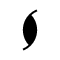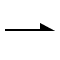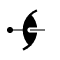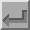Twofold Screw Axis (21)The symbol above shows a twofold screw axis perpendicular to the plane of the screen, i.e parallel to Z. A twofold screw axis is equivalent to a rotation of 180° about a line followed by a translation parallel to that line. A two-one screw axis with a twofold rotation about the c-axis, i.e. about the line 0,0,z, followed by a translation of 1/2 c along it, will have the corresponding symmetry operator -x,-y,1/2+z. This axis has the written symbol "21".The symbol shown above corresponds to a twofold screw axis, but now parallel to the Cartesian X direction. A twofold rotation about the x-axis, followed by a 1/2 a translation along it, will have the corresponding symmetry operator 1/2+x,-y,-z. A fractional value next to the symbol indicates the height of the axis of rotation above the XY plane.In space group diagrams for cubic symmetry, twofold screw axes may appear inclined at an angle of 45° to the XY plane. The symbol for these is shown above, the dot indicating the exact position of intersection with the plane.Back
 © Copyright 1995-2006.  Birkbeck College, University of London. Author(s): Jeremy Karl Cockcroft